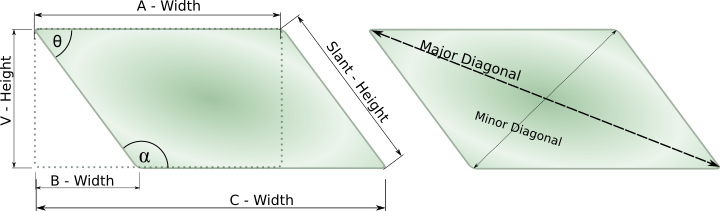# Maths - Parallelograms

Show/Hide:DefinitionsFormulaeInstructions

## Introduction:

This page allows you to calculate every dimension of a Parrallelogram, if you know enough dimensions already. The actual shape that you are interested in can be any physical object whatsoever, possibly a section of lawn in a garden, or any other DIY task.

## Caveats:

If you are reading this paragraph, then you probably don't have JavaScript installed/enabled on your computer.
At this point in time the calculators on this site are entirely dependent on JavaScript. Given enough time and money it is hoped to develop a version in the future which will be able to operate independently. Since JavaScript runs on your own computer, rather than on this server, it has been possible to set up this site much more quickly than if a server-side language was used, and it is possible to serve far more pages with the available bandwidth.
If you think that you can assist with this project in any way, then please visit the Support section and leave a message.

## Definitions:

Parallelogram
A two-dimensional object with four, parallel sides. Opposite sides have the same length, but corners are not right angles (90 degrees.)
A - Width
The distance measured along a full edge of the Parallelogram. In this context which one is considered Length and which one the Width is entirely irrelevant.
B - Width
The distance measured along the extension of one edge of the Parallelogram, as far as the end of the parallel edge. In this context which one is considered Length and which one the Width is entirely irrelevant.
C - Width
The overall distance measured along one edge of the Parallelogram, plus its extension as far as the end of the parallel edge. In this context which one is considered Length and which one the Width is entirely irrelevant.
V - Height
The distance measured vertically (in the diagram) from one side of the Parallelogram to the opposite.
Slant Height
The true length of either of the two sides of the Parallelogram.
Major Diagonal
The distance between the furthest opposite corners of the Parallelogram.
Minor Diagonal
The distance between the closest opposite corners of the Parallelogram.
Circumferance c
The distance measured once around the edge of the Parallelogram.
Area a
The area enclosed by the sides of the Parallelogram.
Angle α (Alpha)
The acute, interior angle between adjacent sides of the Parallelogram.
Angle θ (Theta)
The obtuse, interior angle between adjacent sides of the Parallelogram.

## Formulae:

• c = (2 * l) + (2 * w)
• a = l * w
• d2 = l2 + w2

## Instructions

1. Fill in as many boxes as you can with the values that you know
2. Choose the correct units for all 'input' fields, or leave as 'Units' if not required
3. Choose the correct units for any output fields that you require
4. Click the 'CalcResult' button below the input fields
5. The value of the blank fields will be calculated
6. A 'Results Area' will be displayed below.
7. All known values will be shown. (Values for which insufficient information was given will also be identified.)
8. To do further calculations simply change any values in the input fields and click the 'CalcResult' button again. (Press the 'Clear Form' button if you want to empty all of the input fields.)
9. Press the 'Clear Results' button if you wish to discard the first results, otherwise further calculations will be added into the same area.
10. Click the 'CalcResult' button again
11. Click the 'Print Results' button to display all current results in a new, printable window.